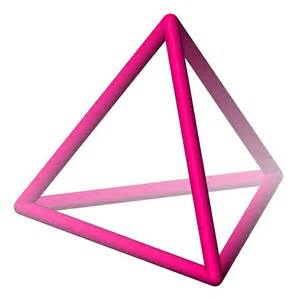# A Neurotic Bug

Probability Level 4

Let $A, B, C$ and $D$ be the vertices of a regular tetrahedron, each of whose edges measures 1 meter. A bug, starting from vertex $A$, observes the following rule:

At each vertex it chooses one of the three edges meeting at that vertex, each edge being equally likely, and crawls along that edge to the vertex at its opposite end.Suddenly the bug remembers that it has left its antenna at $A$.

Find the probability that the bug is at vertex $A$ when it has crawled exactly 7 meters.

If the probability is the form of $\dfrac pq$, where $p$ and $q$ are coprime positive integers, find $p+q$.

×

Problem Loading...

Note Loading...

Set Loading...